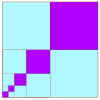# Resources tagged with: Limits of Sequences

Filter by: Content type:
Age range:
Challenge level:

### There are 6 results

Broad Topics > Patterns, Sequences and Structure > Limits of Sequences### Litov's Mean Value Theorem

##### Age 11 to 14Challenge Level

Start with two numbers and generate a sequence where the next number is the mean of the last two numbers...### Archimedes and Numerical Roots

##### Age 14 to 16Challenge Level

The problem is how did Archimedes calculate the lengths of the sides of the polygons which needed him to be able to calculate square roots?### Zooming in on the Squares

##### Age 7 to 14

Start with a large square, join the midpoints of its sides, you'll see four right angled triangles. Remove these triangles, a second square is left. Repeat the operation. What happens?### Continued Fractions I

##### Age 14 to 18

An article introducing continued fractions with some simple puzzles for the reader.### Approximating Pi

##### Age 14 to 18Challenge Level

By inscribing a circle in a square and then a square in a circle find an approximation to pi. By using a hexagon, can you improve on the approximation?### Summing Geometric Progressions

##### Age 14 to 18Challenge Level

Watch the video to see how to sum the sequence. Can you adapt the method to sum other sequences?